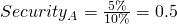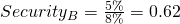# Low volatility portfolio

A low volatility portfolio is a portfolio in which only assets are included that exhibit below average volatility. The meaning of volatility in finance is that less volatile securities are considered less risky. In recent years, a number of low volatility funds, typically low volatility ETFs and low volatility mutual funds, have become available for investing. Low volatility investing has become very popular in recent years.

A low volatility portfolio is considered a type of smart beta. That’s the case because low volatility portfolios are typically not market capitalization weighted, but rather equal-weighted or risk weighted. In that sense, low volatility portfolios are closely related to equal volatility portfolios and risk parity.

## Definition low volatility portfolio

A low volatility fund is a fund that will invest in securities that exhibit lower than average volatility. In other words, it will buy the lowest volatility stocks. The reason this for this is that such portfolios appear to be more attractive from a risk-return perspective than would normally be the case. This, of course, is not consistent with the efficient market hypothesis. That’s why academics talk about the low volatility anomaly. If this sounds confusing, consider a simple example.

## How low volatility portfolios work

To understand, let’s take two securities. Security A has an expected return of 5%, and a volatility of 10%. Security B has an expected return of 5%, but only a volatility of 8%. As such, security B is less volatile, or less risky than security A. In this case, security A is low volatility and security B is high volatility. Now, the risk-return trade-off for both securities is the followingAs we can see, we get more return per ‘unit of risk’ we take. This means that, from a risk-return perspective, the second security is more interesting. Of course, in practice we don’t observe the expected returns, but only observe the securities’ volatility. A low volatility portfolio will just invest in the lowest volatility stocks. Often, the overall volatility of the low volatility portfolio will be quite low, because all the constituent portfolios are very low volatility. In that case, some leverage is applied. This is done to obtain a certain volatility target.

## Low vol strategies in practice

Most low volatility strategies focus on buying low volatility equities. These low volatility equities are then combined into a portfolio using a smart beta weighting approach. This is often equal-weighting or equal-volatility weighting. A low volatility index ETF typically has this structure, i.e. buying the lowest volatility stocks in a certain stock market such as the NYSE or Nasdaq.

One special case of a low volatility portfolio is a “global minimum volatility fund”. Such a portfolio uses as a weighting scheme the minimum variance approach.

## Summary

We discussed low volatility portfolios. These portfolios are based on the finding that low volatility equities outperform high volatility equities. This academic finding is referred to as the low volatility anomaly. It is strongly related to risk-based allocation approaches, such as the equal volatility weighting approach, which can easily be implemented in Excel provided here.